# 目录

## 参考资料

 指数移动平均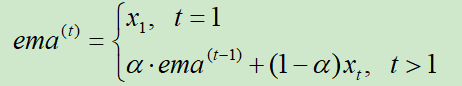import numpy as np
import matplotlib.pyplot as plt
t = [1,2,3,4]
x = [5,10,15,20]
res = [x]
for i in x[1:]:
a = 0.7*res[-1]+0.3*i
res.append(a)
plt.plot(t,x,"r")
plt.plot(t,res,"b")
View Code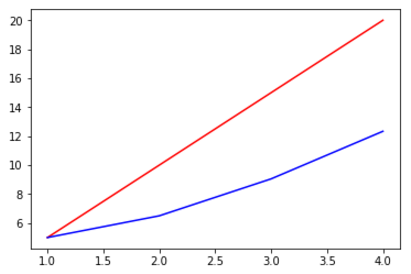import numpy as np
import random
import matplotlib.pyplot as plt
random.seed(20190725)
t = np.linspace(-5,5,100)
x = [-i**2+random.random()*15 for i in t]
res = [x]
for i in x[1:]:
a = 0.7*res[-1]+0.3*i
res.append(a)
plt.plot(t,x,"r")
plt.plot(t,res,"b")
View Code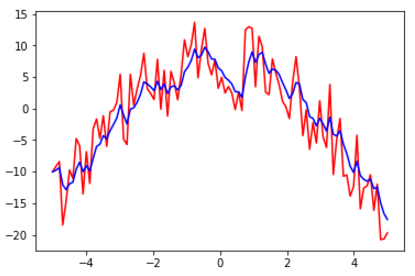BN在卷积网络中的使用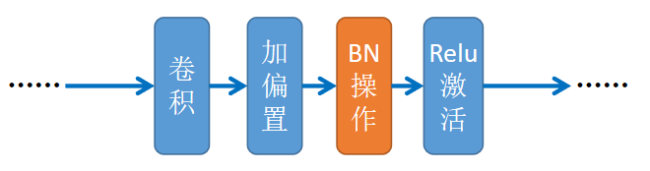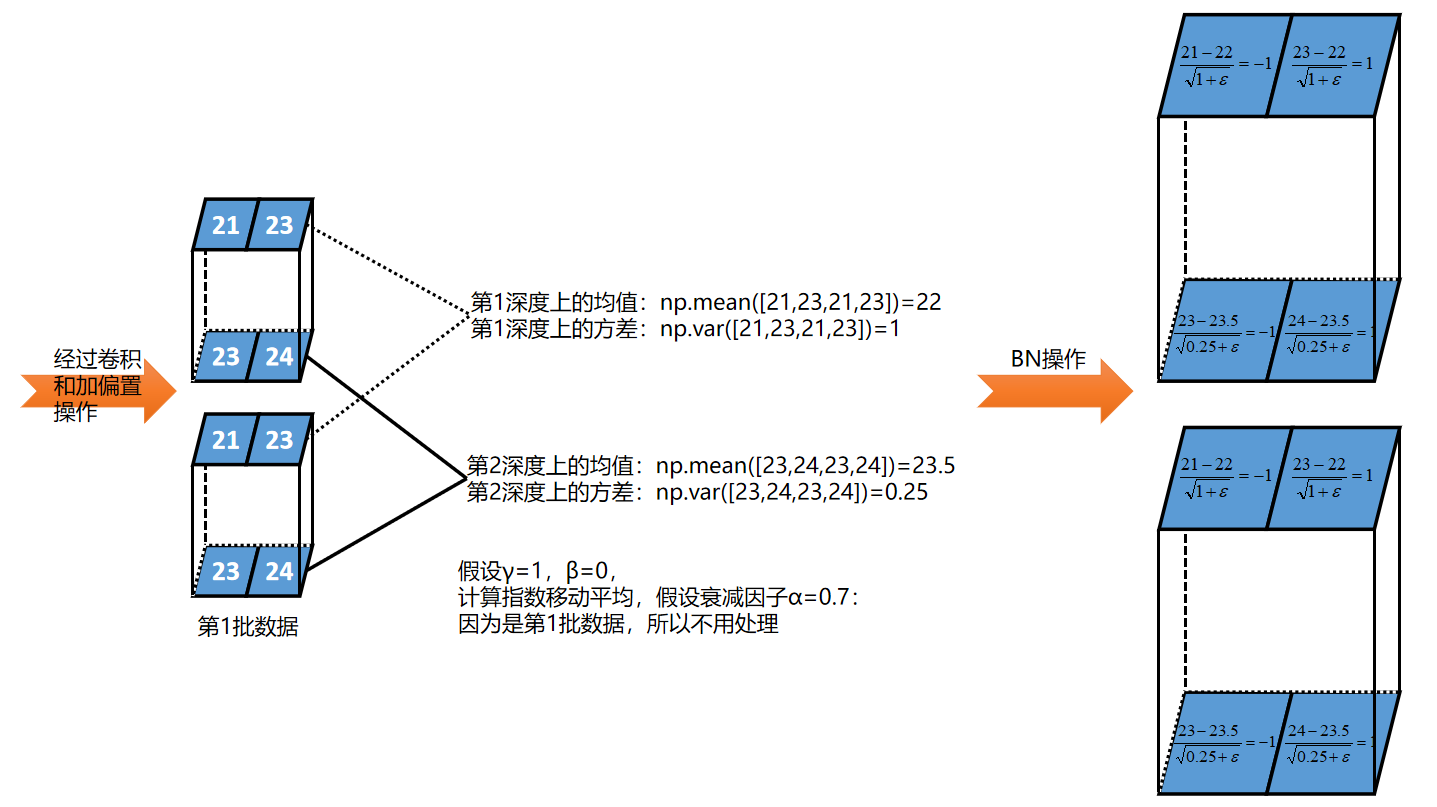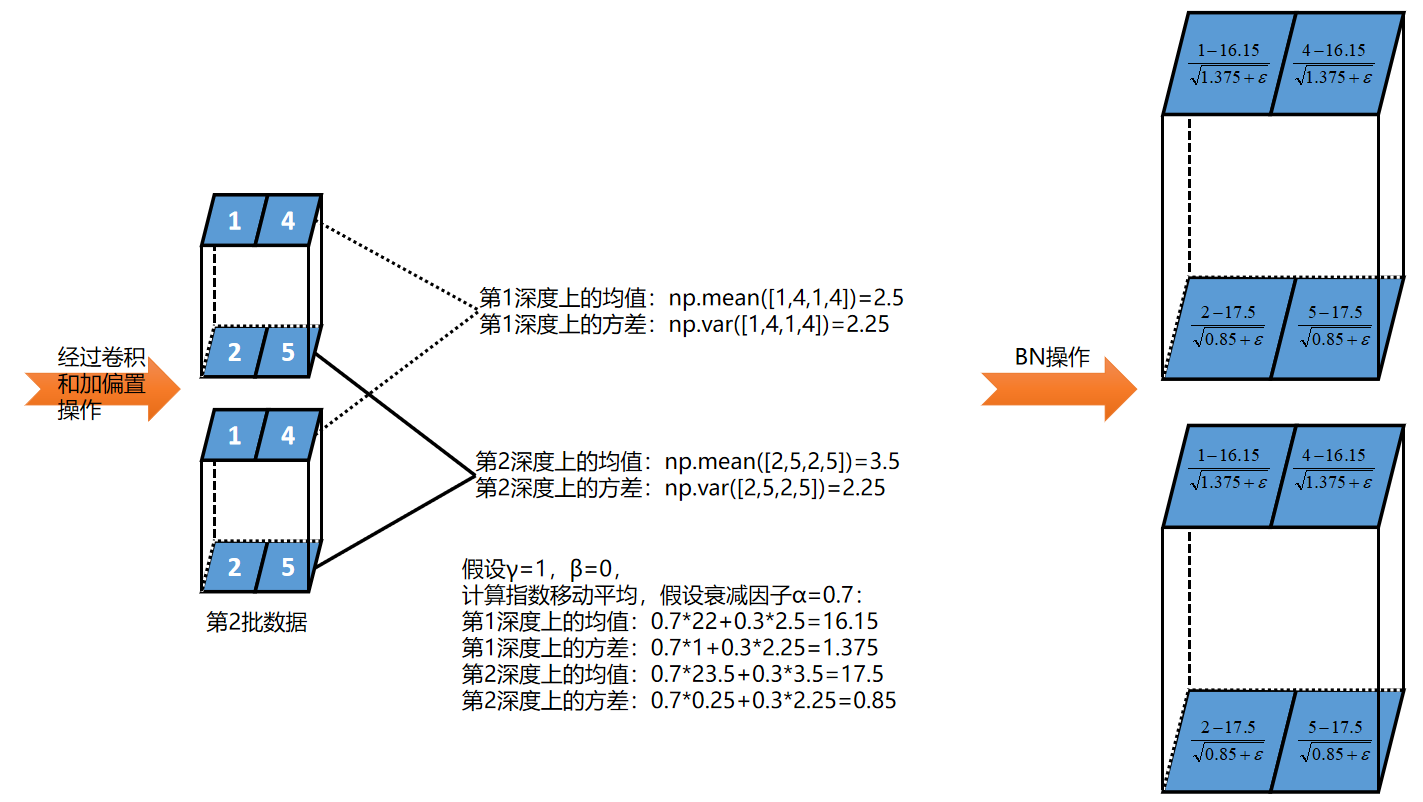参考资料

《图解深度学习与神经网络：从张量到TensorFlow实现》_张平

Batch Normalization_ Accelerating Deep Network Training by Reducing Internal Covariate Shift

posted @ 2019-07-25 11:25  黎明程序员  阅读(601)  评论(0编辑  收藏  举报